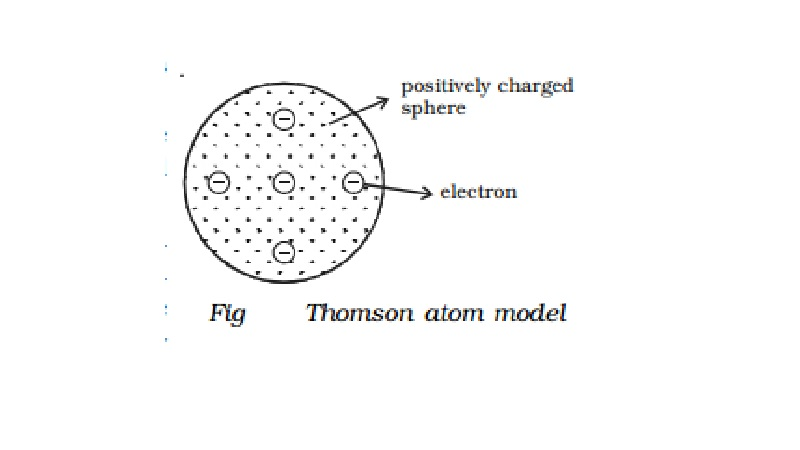Home | | Physics | | Physics | Thomson atom model and its Drawbacks

# Thomson atom model and its DrawbacksAccording to Thomson, if there is a single electron in the atom (like a hydrogen atom), the electron must be situated at the centre of the positive sphere. For an atom with two electrons (helium atom), the electrons should be situated symmetrically with respect to the centre of the sphere i.e., opposite sides of the centre at a distance of r/2 , where r is the radius of the positive sphere.

Thomson atom model

On discharge  of  electricity  through  gases,  it became clear that an atom consists of positive and negative charges. J.J. Thomson tried to explain the arrangement of positive charge and the electrons inside the atom. According to him, an atom is a sphere of positive charge having a radius of the order of 10-10m. The positive charge is uniformly distributed over the entire sphere and the electrons are embedded in the sphere of positive charge as shown in Fig 6.6. The total positive charge inside the atom is equal to the total negative charge carried by the electrons, so that every atom is electrically neutral.

According to Thomson, if there is a single electron in the atom (like a hydrogen atom), the electron must be situated at the centre of the positive sphere. For an atom with two electrons (helium atom), the electrons should be situated symmetrically with respect to the centre of the sphere i.e., opposite sides of the centre at a distance of r/2 , where r is the radius of the positive sphere. In a three electron system of the atom, the electrons should be at the corners of a symmetrically placed equilateral triangle, the side of which was equal to the radius of the sphere. In general, the electrons of an atom are located in a symmetrical pattern with respect to the centre of the sphere.

It was suggested that spectral radiations are due to the simple harmonic motion of these electrons on both sides of their mean positions. Moreover, the stability of the atom was very well explained on the basis of this model.

Drawbacks

i.            According to electromagnetic theory, the vibrating electron should radiate energy and the frequency of the emitted spectral line should be the same as the electron. In the case of hydrogen atom, Thomson's model gives only one spectral line of about 1300 �. But the experimental observations reveal that hydrogen spectrum consists of five different series with several lines in each series.

ii.            It could not account for the scattering of α-particles through large angles.

Study Material, Lecturing Notes, Assignment, Reference, Wiki description explanation, brief detail

Related Topics